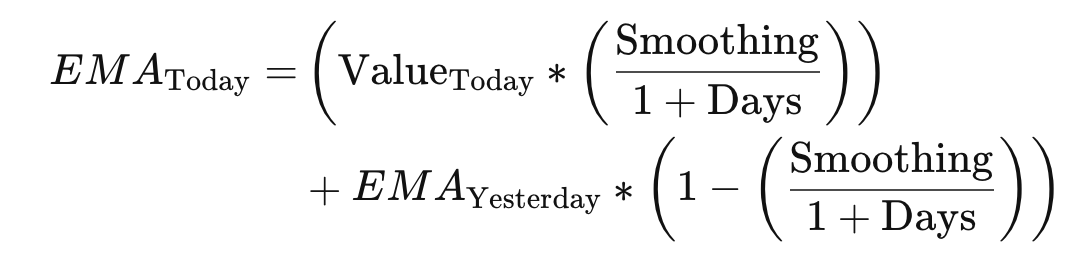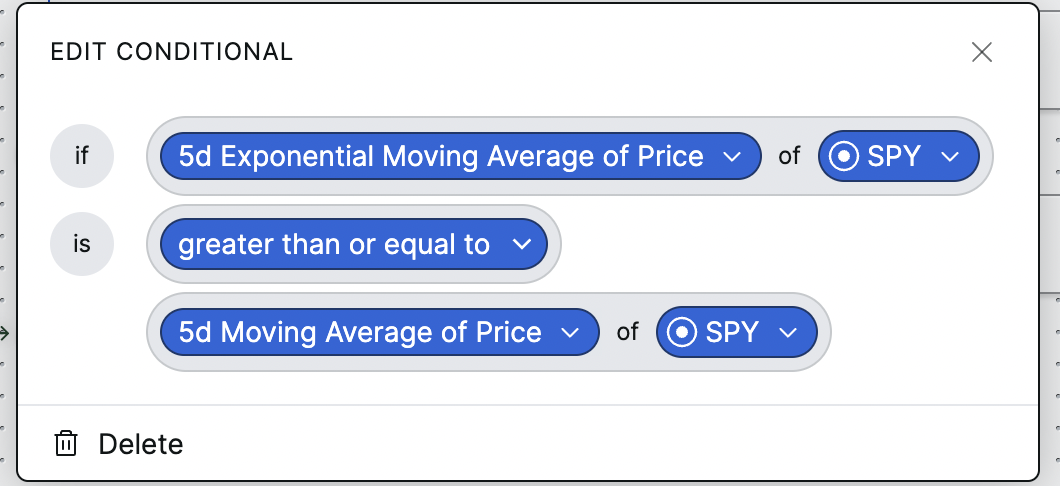# Exponential moving average

In this article, you'll learn about exponential moving average (EMA), how it's calculated, and how it's used in Composer.

What is exponential moving average?

EMA of price is an average price of a selected time period, but with more weight and significance placed on recent datapoints. The amount of weight given to recent results is called the "smoothing factor" with the most commonly used smoothing factor being 2.0. This is what Composer uses.

Formula

The formula for exponential moving average is as follows:Example

In our piece on simple moving average, we used an example of an asset that increased by \$1 every day of a week, as follows:

• Monday: \$6
• Tuesday: \$7
• Wednesday: \$8
• Thursday: \$9
• Friday: \$10

The 5-day simple moving average for this asset would be calculated as \$8.00. Using a smoothing factor of 2, the 5-day EMA would be calculated as \$9.33, giving more weight to Thursday and Friday's values.

Where is EMA in Composer?Exponential moving average can be used in conditional and filter blocks. In the example above, we'd take the 5-day EMA of SPY, and compare that to the 5-day SMA of SPY. The exponential moving average could signal a recent upswing, given its weighting towards recent data. If the 5-day EMA of SPY is greater than or equal to the 5-day SMA of SPY, the blocks underneath the "IF" would execute, while if the EMA was less than the SMA, the blocks underneath the "ELSE" function would execute.